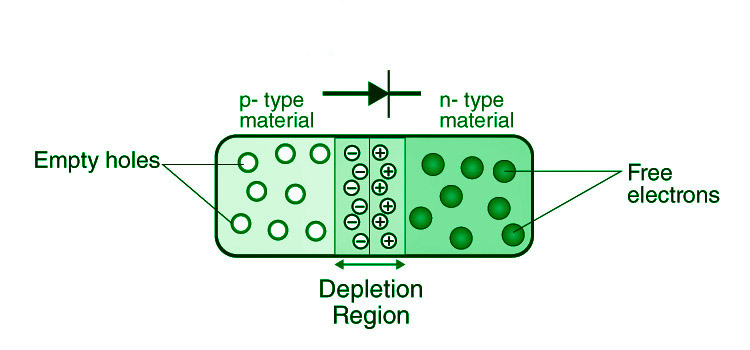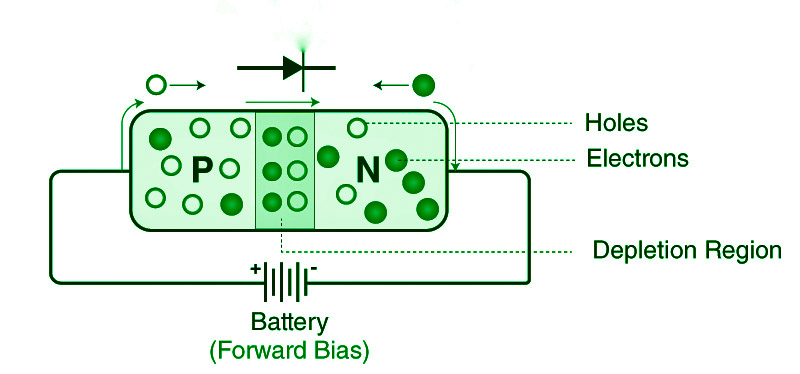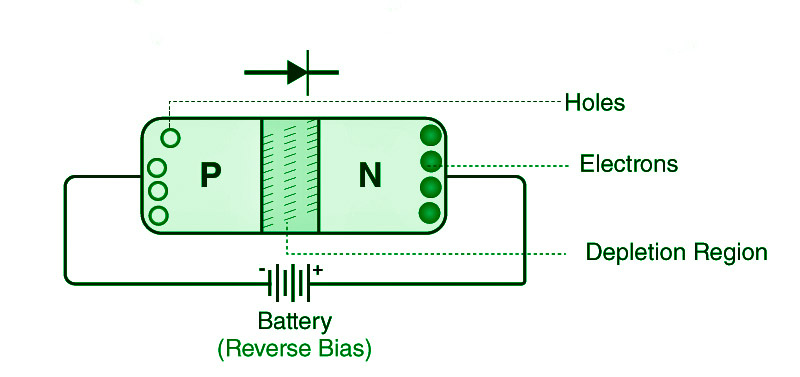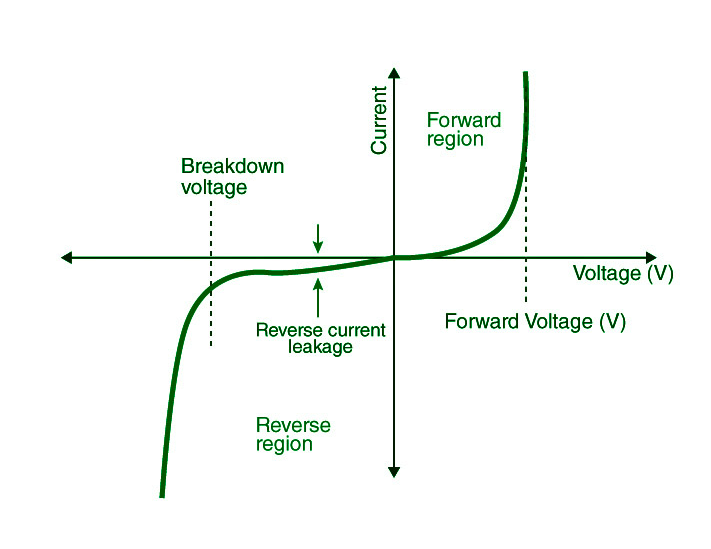Open in App
Not now

## Related Articles

• CBSE Class 12 Syllabus
• CBSE Class 12 Maths Notes
• CBSE Class 12 Physics Notes
• CBSE Class 12 Chemistry Notes
• CBSE Class 12 Accountancy Notes
• CBSE Class 12 Computer Science (Self-Paced Course)

# p-n Junction Diode

• Difficulty Level : Basic
• Last Updated : 08 Nov, 2022

The electrical conductivity of a semiconductor material is between that of a conductor, such as metallic copper, and that of an insulator, such as glass. Its resistivity decreases as the temperature rises, whereas metals have the reverse effect. By adding impurities (doping) into the crystal structure, its conducting characteristics can be changed in beneficial ways. A semiconductor junction is formed when two distinct doped areas occur in the same crystal. Diodes, transistors, and most contemporary electronics are built on the behaviour of charge carriers such as electrons, ions, and electron holes at these junctions.

Silicon, germanium, gallium arsenide, and elements along the periodic table’s so-called metalloid staircase are examples of semiconductors. Gallium arsenide is the second most common semiconductor after silicon, and it is used in laser diodes, solar cells, microwave-frequency integrated circuits, and other applications. Silicon is a crucial component in the production of most electrical circuits.

### p-n Junction

Inside a semiconductor, a p-n junction is an interface or a border between two semiconductor material types, namely the p-type and the n-type.

The semiconductor’s p-side, or positive side, has an excess of holes, whereas the n-side, or negative side, has an excess of electrons. The doping process is used to produce the p-n junction in a semiconductor.

### Formation of P-N Junction

When we utilise various semiconductor materials to form a p-n junction, there will be a grain boundary that will prevent electrons from moving from one side to the other by scattering electrons and holes, which is why we employ the doping procedure.

For example, Consider a p-type silicon semiconductor sheet that is very thin. A portion of the p-type Si will be changed to n-type silicon if a tiny quantity of pentavalent impurity is added. This sheet will now have both a p-type and an n-type area, as well as a junction between the two. Diffusion and drift are the two sorts of processes that occur following the creation of a p-n junction. As we all know, the concentration of holes and electrons on the two sides of a junction differs, with holes from the p-side diffusing to the n-side and electrons from the n-side diffusing to the p-side. This causes a diffusion current to flow across the connection.When an electron diffuses from the n-side to the p-side, it leaves an ionised donor on the n-side, which is stationary. On the n-side of the junction, a layer of positive charge develops as the process progresses. When a hole is moved from the p-side to the n-side, an ionised acceptor is left behind on the p-side, causing a layer of negative charges to develop on the p-side of the junction. The depletion area is defined as a region of positive and negative charge on each side of the junction. An electric field direction from a positive charge to a negative charge is generated due to this positive space charge area on each side of the junction. An electron on the p-side of the junction travels to the n-side of the junction due to the electric field. The drift is the name given to this motion. We can observe that the drift current runs in the opposite direction as the diffusion current.

### Biasing conditions for the p-n Junction Diode

In a p-n junction diode, there are two operational regions:

1. p-type
2. n-type

The voltage applied determines one of three biasing conditions for p-n junction diodes:

• There is no external voltage provided to the p-n junction diode while it is at zero bias.
• Forward bias: The p-type is linked to the positive terminal of the voltage potential, while the n-type is connected to the negative terminal.
• Reverse bias: The p-type is linked to the negative terminal of the voltage potential, while the n-type is connected to the positive terminal.

### Forward Bias

The p-n junction is said to be forward-biased when the p-type is connected to the positive terminal of the battery and the n-type to the negative terminal. The built-in electric field at the p-n junction and the applied electric field are in opposing directions when the p-n junction is forward biased.

The resulting electric field is smaller than the built-in electric field when both electric fields are added together. As a result, the depletion area becomes less resistant and thinner. When the applied voltage is high, the resistance of the depletion zone becomes insignificant. At 0.6 V, the resistance of the depletion area in silicon becomes absolutely insignificant, allowing current to flow freely over it.### Reverse Bias

The p-n junction is said to be reverse-biased when the p-type is linked to the negative terminal of the battery and the n-type is attached to the positive side. The applied electric field and the built-in electric field are both in the same direction in this situation.

The resultant electric field is in the same direction as the built-in electric field, resulting in a more resistive, thicker depletion zone. If the applied voltage is increased, the depletion area gets more resistant and thicker.### P-N Junction Formula

The p-n junction formula, which is based on the built-in potential difference generated by the electric field, is as follows:

Eo= VTln [ ND – NA / ni2 ]

where,

• The zero-bias junction voltage is E0.
• At room temperature, VT is the thermal voltage of 26 mV.
• The impurity concentrations are denoted by the letters ND and NA.
• The intrinsic concentration is denoted by n

### Flow of current in p-n junction diode

When the voltage is increased, electrons move from the n-side to the p-side of the junction. The migration of holes from the p-side to the n-side of the junction occurs in a similar manner as the voltage rises. As a result, a concentration gradient exists between the terminals on both sides.

There will be a movement of charge carriers from higher concentration regions to lower concentration regions as a result of the development of the concentration gradient. The current flow in the circuit is caused by the movement of charge carriers inside the p-n junction.

### V-I Characteristics of PN Junction DiodeA curve between the voltage and current across the circuit defines the V-I properties of p-n junction diodes. The x-axis represents voltage, while the y-axis represents current. The V-I characteristics curve of the p-n junction diode is shown in the graph above. With the help of the curve, we can see that the diode works in three different areas, which are:

• Zero bias
• Forward bias
• Reverse bias

There is no external voltage provided to the p-n junction diode while it is at zero bias, which implies the potential barrier at the junction prevents current passage.

When the p-n junction diode is in forwarding bias, the p-type is linked to the positive terminal of the external voltage, while the n-type is connected to the negative terminal. The potential barrier is reduced when the diode is placed in this fashion. When the voltage is 0.7 V for silicon diodes and 0.3 V for germanium diodes, the potential barriers fall and current flows.

The current grows slowly while the diode is under forwarding bias, and the curve formed is non-linear as the voltage supplied to the diode overcomes the potential barrier. Once the diode has crossed the potential barrier, it functions normally, and the curve rises quickly as the external voltage rises, yielding a linear curve.

When the PN junction diode is under negative bias, the p-type is linked to the negative terminal of the external voltage, while the n-type is connected to the positive terminal. As a result, the potential barrier becomes higher. Because minority carriers are present at the junction, reverse saturation current occurs at first.

When the applied voltage is raised, the kinetic energy of the minority charges increases, affecting the majority charges. This is the point at which the diode fails. The diode may be destroyed as a result of this.

### Applications of PN Junction Diode

• When the p-n junction diode’s arrangement is reverse-biased, the diode may be utilised as a photodiode since it is sensitive to light.
• It has the potential to be utilised as a solar cell.
• The diode can be utilised in LED lighting applications when it is forward-biased.
• Many electric circuits utilise it as a rectifier, while varactors employ it as a voltage-controlled oscillator.

### Sample Question

Question 1: What is reverse resistance?

The resistance supplied by a p-n junction diode when it is reverse biased is known as reverse resistance.

Question 2: What is a diode’s dynamic resistance?

The ratio of change in voltage to change in current is known as dynamic resistance of a diode.

Question 3: What is a diode’s static resistance?

The ratio of the DC voltage put across the diode to the DC current flowing through it is known as the diode’s static resistance.

Question 4: What is reverse bias?